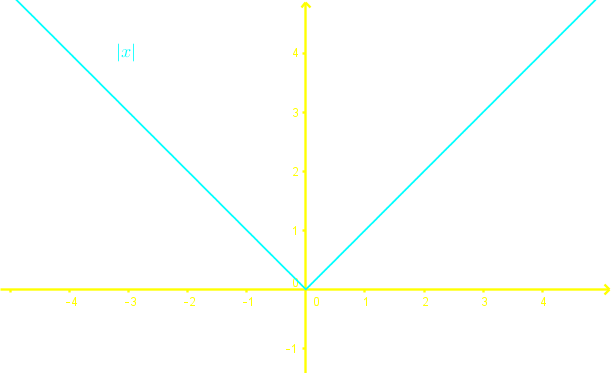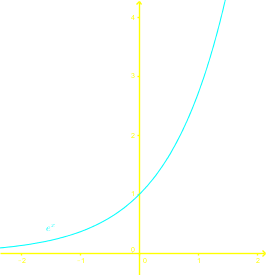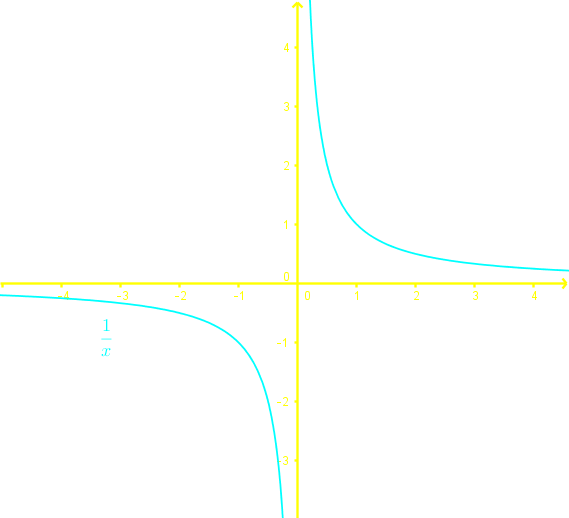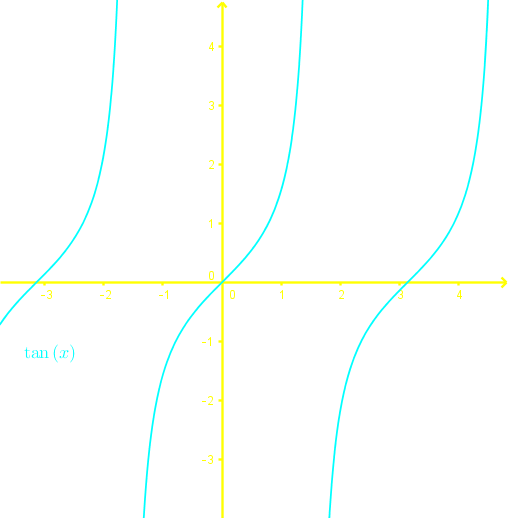# One to one functions

### One to one functions

Basic Concepts: Inverse functions

#### Lessons

$\bullet$ To determine if an expression is a function, we perform the vertical line test.

$\bullet$ Surjective/Onto: For every $y$ value, there exists at least one $x$ value.

$\bullet$ Injective/Into/one-to-one: For every $y$ value, there exists at most one $x$ value.

$\bullet$ To determine if a function is one-to-one, we perform the horizontal line test.

• Introduction
Introduction to one to one functions

i. Review: How are functions, Surjective functions and Injective functions related?

ii. How to determine if an expression is a function?

iii. What are Surjective functions?

iv. What are one to one functions?

• 1.
Discussing the Differences Between Surjective and Injective Functions

Identify the differences between Surjective and Injective functions and give an example for each of the functions.

• 2.
Identifying One-to-One Functions On a Graph

Learning the Horizontal Line Test and understanding how it can be implemented to identify one-to-one functions on a graph.

• 3.
Applying the Horizontal Line Test

Determine if the following graphs are one-to-one functions using the horizontal line test.

i.ii.iii.iv.